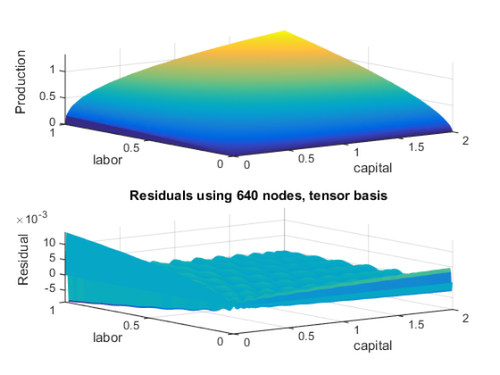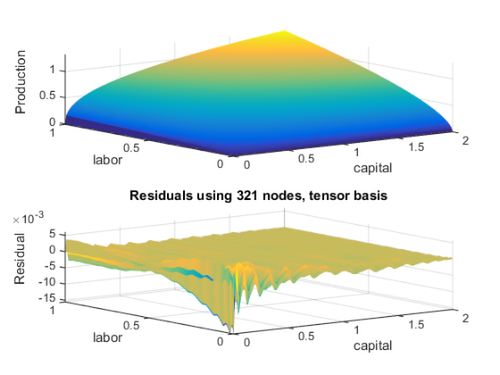# DEMO: basis

This script provides examples on using the basisChebyshev class.

Last updated: October 4, 2014.

## EXAMPLE 2: Using basis and funcApprox to approximate a CobbDouglas function

PROBLEM: Approximate the Cobb-Douglas production function y = f(K,L) = K^0.4 L^0.6 for capital K in [0,2] and labor L in [0,1].

• First, create the production function
```y = @(K,L) K.^0.4 .* L.^0.6;
```

## Using a tensor-product basis

Create a 2-D Chebyshev basis T by taking the tensor product of the individual dimensions

```opts.type = 'cheb';
opts.method = 'tensor';
opts.nodetype = 'lobatto';
opts.varnames = {'capital','labor'};

T = basis([32 20],[0 0],[2 1],opts);
disp(T)
```
```Basis of 2 dimension(s)

Variable           # of nodes       Interval
capital                    32       [  0.00,   2.00]
labor                      20       [  0.00,   1.00]

Type of basis:         cheb
Type of nodes:         lobatto
Expansion method:      tensor
Total basis nodes:     640
Total basis functions: 640

```

The level of production at the collocation nodes is

```yTnodes = y(T.nodes(:,1),T.nodes(:,2));
```

The approximated function, using basis T, is

```yT = funcApprox(T,yTnodes);
```

Plot the functions

```ngrid = 201;
kk = linspace(T.a(1),T.b(1),ngrid)';
ll = linspace(T.a(2),T.b(2),ngrid)';
[kk,ll] = meshgrid(kk,ll);

yy = y(kk,ll);
yTapprox = reshape(yT.Interpolate([kk(:),ll(:)]),ngrid,ngrid);

figure
subplot(2,1,1), surf(kk,ll,yy,'EdgeColor','none')
hold on
surf(kk,ll,yTapprox,'EdgeColor','none')
axis tight
xlabel(T.opts.varnames(1)), ylabel(T.opts.varnames(2)), zlabel('Production')

subplot(2,1,2), surf(kk,ll,yTapprox - yy,'EdgeColor','none')
title('Residuals using 640 nodes, tensor basis'), axis tight
xlabel(T.opts.varnames(1)), ylabel(T.opts.varnames(2)), zlabel('Residual')
```## Using a Smolyak basis

Now repeat the exercise with Smolyak nodes, with degree and node parameters set to q = 3. Call the basis S

```opts.method = 'smolyak';
opts.degreeParam = 6;
opts.nodeParam = 6;

S = basis([80 50],[0 0],[2 1],opts);
disp(S)
```

Notice the warning about the number of Smolyak nodes: The number of nodes for each dimension must be n = 2^k+1 for some positive integer k. If user calls the constructor with different number of nodes, the constructor increases n to the next admissible value (from 80 to 129 for capital, and from 50 to 65 for labor).

```Warning: For Smolyak expansion, number of nodes should be n=2^k + 1,for some k =
1,2,...
Old n,  New n
80,    129
50,     65

Basis of 2 dimension(s)

Variable           # of nodes       Interval
capital                    129      [  0.00,   2.00]
labor                      65       [  0.00,   1.00]

Type of basis:         cheb
Type of nodes:         lobatto
Expansion method:      smolyak
Total basis nodes:     321
Total basis functions: 321

```

The level of production at the new collocation nodes is

```ySnodes = y(S.nodes(:,1),S.nodes(:,2));
```

The approximated function, using basis S, is

```yS = funcApprox(S,ySnodes);
```

Plot the functions

```ySapprox = reshape(yS.Interpolate([kk(:),ll(:)]),ngrid,ngrid);

figure
subplot(2,1,1), surf(kk,ll,yy,'EdgeColor','none')
hold on
surf(kk,ll,ySapprox,'EdgeColor','none')
axis tight
xlabel(S.opts.varnames(1)), ylabel(S.opts.varnames(2)), zlabel('Production')

subplot(2,1,2), surf(kk,ll,ySapprox - yy,'EdgeColor','none')
title('Residuals using 321 nodes, tensor basis'), axis tight
xlabel(S.opts.varnames(1)), ylabel(S.opts.varnames(2)), zlabel('Residual')
```Notice that the residuals in Smolyak and tensor bases are of similar magnitude, despite the fact that Smolyak is using around half the number of nodes and bases functions (i.e., its interpolation matrix is around one-forth the size of the tensor interpolating matrix).